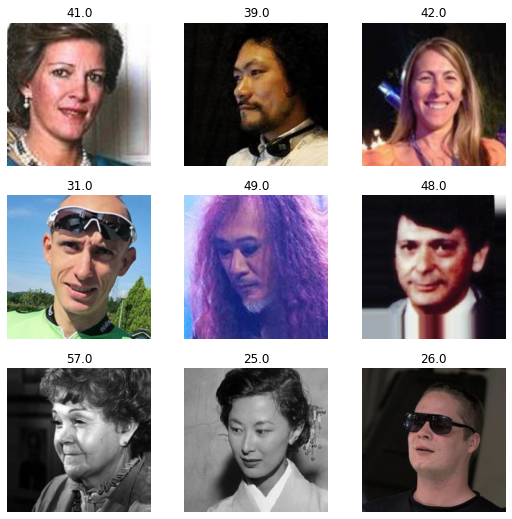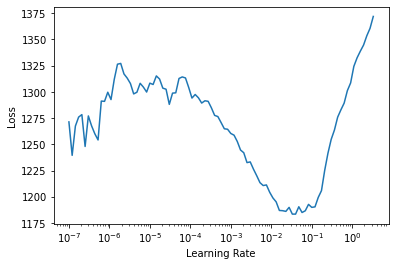This article is also a Jupyter Notebook available to be run from the top down. There will be code snippets that you can then run in any environment.

Below are the versions of fastai, fastcore, and wwf currently running at the time of writing this:

• fastai: 2.1.10
• fastcore: 1.3.13
• wwf: 0.0.7

This notebook describes how to perform Scalar regression on images

Importing the library

Today will be a vision based task:

from fastai.vision.all import *

Below you will find the exact imports for everything we use today

from fastcore.basics import Int
from fastcore.transform import DisplayedTransform
from fastcore.xtras import Path

from fastai.callback.progress import ProgressCallback
from fastai.callback.schedule import fine_tune

from fastai.data.block import DataBlock, RegressionBlock
from fastai.data.transforms import RandomSplitter, get_image_files, ColReader

from fastai.losses import MSELossFlat

from fastai.torch_core import ShowTitle, show_title, TitledFloat

from fastai.vision.augment import Resize, aug_transforms, Flip, Rotate, Zoom, Warp
from fastai.vision.data import ImageBlock
from fastai.vision.learner import cnn_learner

from torchvision.models.resnet import resnet50

from datetime import datetime, timedelta
import pandas as pd

import numpy as np

Dataset

The dataset is the IMDB-WIKI dataset in which we try to guess the expected age from an indivudal image. We'll focus on the WIKI dataset.

url = 'https://data.vision.ee.ethz.ch/cvl/rrothe/imdb-wiki/static/wiki_crop.tar'
!wget {url}
--2020-12-31 17:06:00--  https://data.vision.ee.ethz.ch/cvl/rrothe/imdb-wiki/static/wiki_crop.tar
Resolving data.vision.ee.ethz.ch (data.vision.ee.ethz.ch)... 129.132.52.162
Connecting to data.vision.ee.ethz.ch (data.vision.ee.ethz.ch)|129.132.52.162|:443... connected.
HTTP request sent, awaiting response... 200 OK
Length: 811315200 (774M) [application/x-tar]
Saving to: ‘wiki_crop.tar’

wiki_crop.tar       100%[===================>] 773.73M  19.6MB/s    in 41s

2020-12-31 17:06:42 (18.9 MB/s) - ‘wiki_crop.tar’ saved [811315200/811315200]

!tar -xvf 'wiki_crop.tar'

Now the dataset itself is pretty easy, there is an included .mat file we can convert to Pandas. Let's go through that process:

We are following the same preperation code as seen here

• Note: the video and this notebook differ here. This is because I was labelling the data wrong intially, and the floats are now inheritly supported
from datetime import datetime, timedelta
def prepare_dataframe(path:Path):
"Builds wiki_crop DataFrame based on https://sefiks.com/2019/02/13/apparent-age-and-gender-prediction-in-keras/"
instances = mat['wiki'].shape
columns = ['dob', 'photo_taken', 'full_path', 'gender', 'name', 'face_location', 'face_score', 'second_face_score']
df = pd.DataFrame(index=range(0, instances), columns=columns)
for i in mat:
if i == 'wiki':
curr_arr = mat[i]
for j, val in enumerate(curr_arr):
df[columns[j]] = pd.DataFrame(val)

### Python datatime conversion
df['date_of_birth'] = df['dob'].apply(datenum_to_datetime)
df['age'] = df['photo_taken'] - df['date_of_birth']

## Data cleaning
df = df[df['face_score'] != -np.inf] # picture doesn't include faces
df = df[df['second_face_score'].isna()] # more than one face
df = df[df['face_score'] > 3] # has a score
df = df[df['age'] < 100] # older than 100 (mostly paintings)
df = df[df['age'] > 0] # unborn people
df = df[['full_path', 'age']]
df['full_path'] = df['full_path'].apply(lambda x: x)
return df

def datenum_to_datetime(datenum):
days = datenum % 1
hours = days % 1 * 24
minutes = hours % 1 * 60
seconds = minutes % 1 * 60
exact_date = datetime.fromordinal(int(datenum)) \
+ timedelta(days=int(days)) + timedelta(hours=int(hours)) \
+ timedelta(minutes=int(minutes)) + timedelta(seconds=round(seconds)) \
- timedelta(days=366)
return exact_date.year
path = Path('wiki_crop/wiki.mat')
df = prepare_dataframe(path)
full_path age
0 17/10000217_1981-05-05_2009.jpg 28
2 12/100012_1948-07-03_2008.jpg 60
4 16/10002116_1971-05-31_2012.jpg 41
5 02/10002702_1960-11-09_2012.jpg 52
6 41/10003541_1937-09-27_1971.jpg 34

Let's make a get_x and a get_y since we are using a DataFrame

block = DataBlock(blocks=(ImageBlock, RegressionBlock()),
get_x = get_x,
get_y = get_y,
splitter=RandomSplitter(),
item_tfms=Resize(460, method='squish'),
batch_tfms=[*aug_transforms(size=224, max_warp=0)])

Let's look at a batch of data;

dls.show_batch()dls.c
1

And now we can run this through cnn_learner

from fastai.metrics import mae
learn = cnn_learner(dls, resnet50, loss_func=MSELossFlat(), y_range=(0,100), metrics=mae)